# Profit Margin

Calculating gross profit margin, operating profit margin and net profit margin in Excel is easy. Simply use the formulas explained on this page.

### Gross Profit Margin

Assume your business had a total revenue of \$10,000 in July and the cost of goods sold (COGS) equaled \$4,000.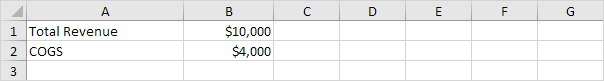To calculate the gross profit margin (GPM), use the following formula:

 Gross Profit MarginGPM = Total Revenue - COGS Total Revenue

1. The formula below calculates the number above the fraction line. This is called the gross profit.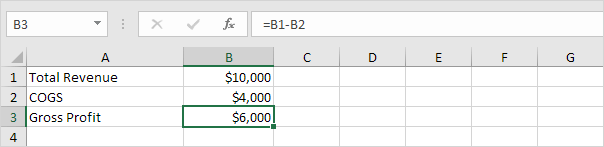2. Divide this result by the total revenue to calculate the gross profit margin in Excel.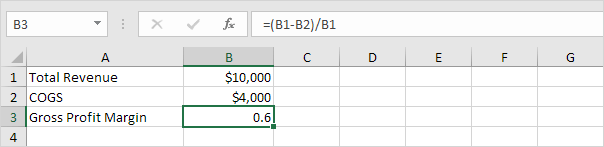3. On the Home tab, in the Number group, click the percentage symbol to apply a Percentage format.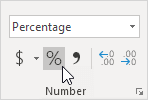Result: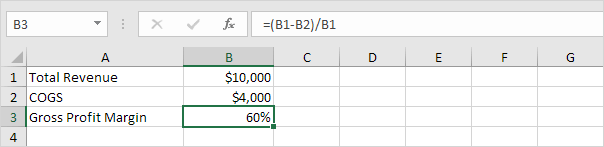### Operating Profit Margin

The operating profit margin also includes operating expenses (OPEX) such as rent, equipment, inventory costs, marketing, etc.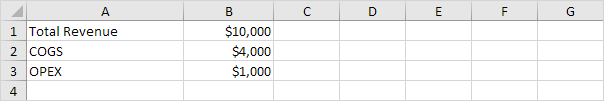To calculate the operating profit margin (OPM), use the following formula:

 Operating Profit MarginOPM = Total Revenue - COGS - OPEX Total Revenue

1. The formula below calculates the number above the fraction line. This is called the operating profit.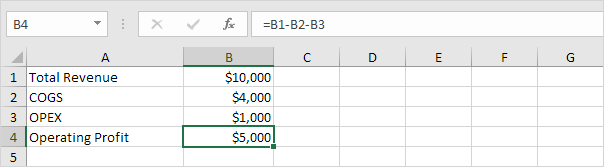2. Divide this result by the total revenue to calculate the operating profit margin in Excel.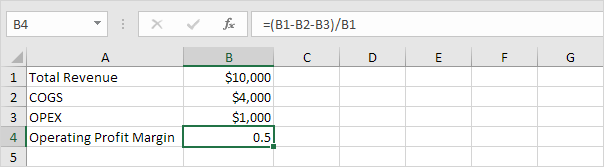3. On the Home tab, in the Number group, click the percentage symbol to apply a Percentage format.Result: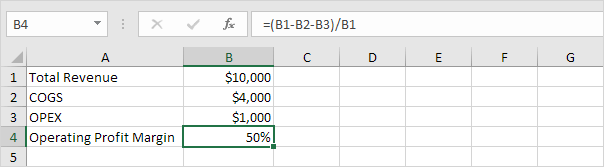### Net Profit Margin

The net profit margin also includes non-operating expenses such as interest (on debt) and taxes.To calculate the net profit margin (NPM), use the following formula:

 Net Profit MarginNPM = Total Revenue - COGS - OPEX - I - T Total Revenue

where:

COGS = Cost of Goods Sold

OPEX = Operating expenses

I = Interest

T = Taxes

1. The formula below calculates the number above the fraction line. This is called the net income.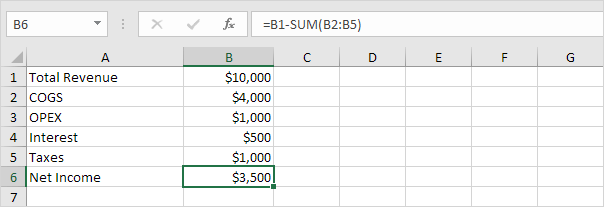2. Divide this result by the total revenue to calculate the net profit margin in Excel.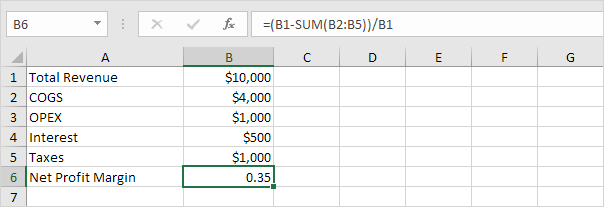3. On the Home tab, in the Number group, click the percentage symbol to apply a Percentage format.Result:Conclusion: a 35% net profit margin means your business has a net income of \$0.35 for each dollar of sales.

Go to Next Chapter: Statistical Functions